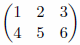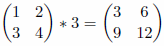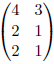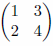Matrix Calculator

Time Limit : 8 sec, Memory Limit : 131072 KB

Problem J: Matrix Calculator

Dr. Jimbo, an applied mathematician, needs to calculate matrices all day for solving his own problems. In his laboratory, he uses an excellent application program for manipulating matrix expressions, however, he cannot use it outside his laboratory because the software consumes much of resources. He wants to manipulate matrices outside, so he needs a small program similar to the excellent application for his handheld computer.

Your job is to provide him a program that computes expressions of matrices.

Expressions of matrices are described in a simple language. Its syntax is shown in Table J.1. Note that even a space and a newline have meaningful roles in the syntax.The start symbol of this syntax is program that is defined as a sequence of assignments in Table J.1. Each assignment has a variable on the left hand side of an equal symbol ("=") and an expression of matrices on the right hand side followed by a period and a newline (NL). It denotes an assignment of the value of the expression to the variable. The variable (var in Table J.1) is indicated by an uppercase Roman letter. The value of the expression (expr) is a matrix or a scalar, whose elements are integers. Here, a scalar integer and a 1 × 1 matrix whose only element is the same integer can be used interchangeably.

An expression is one or more terms connected by "+" or "-" symbols. A term is one or more factors connected by "*" symbol. These operators ("+", "-", "*") are left associative.

A factor is either a primary expression (primary) or a "-" symbol followed by a factor. This unary operator "-" is right associative.

The meaning of operators are the same as those in the ordinary arithmetic on matrices: Denoting matrices by A and B, A + B, A - B, A * B, and -A are defined as the matrix sum, difference, product, and negation. The sizes of A and B should be the same for addition and subtraction. The number of columns of A and the number of rows of B should be the same for multiplication.

Note that all the operators +, -, * and unary - represent computations of addition, subtraction, multiplication and negation modulo M = 215 = 32768, respectively. Thus all the values are nonnegative integers between 0 and 32767, inclusive. For example, the result of an expression 2 - 3 should be 32767, instead of -1.

inum is a non-negative decimal integer less than M.

var represents the matrix that is assigned to the variable var in the most recent preceding assignment statement of the same variable.

matrix represents a mathematical matrix similar to a 2-dimensional array whose elements are integers. It is denoted by a row-seq with a pair of enclosing square brackets. row-seq represents a sequence of rows, adjacent two of which are separated by a semicolon. row represents a sequence of expressions, adjacent two of which are separated by a space character.

For example, [1 2 3;4 5 6] represents a matrix. The first row has three integers separated by two space characters, i.e. "1 2 3". The second row has three integers, i.e. "4 5 6". Here, the row-seq consists of the two rows separated by a semicolon. The matrix is denoted by the row-seq with a pair of square brackets.

Note that elements of a row may be matrices again. Thus the nested representation of a matrix may appear. The number of rows of the value of each expression of a row should be the same, and the number of columns of the value of each row of a row-seq should be the same.

For example, a matrix represented by

```             [[1 2 3;4 5 6] [7 8;9 10] [11;12];13 14 15 16 17 18]
```

isThe sizes of matrices should be consistent, as mentioned above, in order to form a well-formed matrix as the result. For example, [[1 2;3 4] [5;6;7];6 7 8] is not consistent since the first row "[1 2;3 4] [5;6;7]" has two matrices (2 × 2 and 3 × 1) whose numbers of rows are different. [1 2;3 4 5] is not consistent since the number of columns of two rows are different.

The multiplication of 1 × 1 matrix and m × n matrix is well-defined for arbitrary m > 0 and n > 0, since a 1 × 1 matrices can be regarded as a scalar integer. For example, 2*[1 2;3 4] and [1 2;3 4]*3 represent the products of a scalar and a matrixand. *[1 2;3 4] and [1 2;3 4]* are also well-defined similarly.

An indexed-primary is a primary expression followed by two expressions as indices. The first index is 1 × k integer matrix denoted by (i1 i2 ... ik), and the second index is 1 × l integer matrix denoted by (j1 j2 ... jl). The two indices specify the submatrix extracted from the matrix which is the value of the preceding primary expression. The size of the submatrix is k × l and whose (a, b)-element is the (ia, jb)-element of the value of the preceding primary expression. The way of indexing is one-origin, i.e., the first element is indexed by 1.

For example, the value of ([1 2;3 4]+[3 0;0 2])(,) is equal to 2, since the value of its primary expression is a matrix [4 2;3 6], and the first index  and the second  indicate the (1, 2)-element of the matrix. The same integer may appear twice or more in an index matrix, e.g., the first index matrix of an expression [1 2;3 4]([2 1 1],[2 1]) is [2 1 1], which has two 1's. Its value is.

A transposed-primary is a primary expression followed by a single quote symbol ("'"), which indicates the transpose operation. The transposed matrix of an m × n matrix A = (aij) (i = 1, ..., m and j = 1, ... , n) is the n × m matrix B = (bij) (i = 1, ... , n and j = 1, ... , m), where bij = aji. For example, the value of [1 2;3 4]' is.

Input

The input consists of multiple datasets, followed by a line containing a zero. Each dataset has the following format.

n
program

n is a positive integer, which is followed by a program that is a sequence of single or multiple lines each of which is an assignment statement whose syntax is defined in Table J.1. n indicates the number of the assignment statements in the program. All the values of vars are undefined at the beginning of a program.

You can assume the following:

• 1 ≤ n ≤ 10,
• the number of characters in a line does not exceed 80 (excluding a newline),
• there are no syntax errors and semantic errors (e.g., reference of undefined var),
• the number of rows of matrices appearing in the computations does not exceed 100, and
• the number of columns of matrices appearing in the computations does not exceed 100.

Output

For each dataset, the value of the expression of each assignment statement of the program should be printed in the same order. All the values should be printed as non-negative integers less than M.

When the value is an m × n matrix A = (aij) (i = 1, ... ,m and j = 1, ... , n), m lines should be printed. In the k-th line (1 ≤ km), integers of the k-th row, i.e., ak1, ... , akn, should be printed separated by a space.

After the last value of a dataset is printed, a line containing five minus symbols '-----' should be printed for human readability.

The output should not contain any other extra characters.

Sample Input

```1
A=[1 2 3;4 5 6].
1
A=[[1 2 3;4 5 6] [7 8;9 10] [11;12];13 14 15 16 17 18].
3
B=[3 -2 1;-9 8 7].
C=([1 2 3;4 5 6]+B)(2,3).
D=([1 2 3;4 5 6]+B)([1 2],[2 3]).
5
A=2*[1 2;-3 4]'.
B=A([2 1 2],[2 1]).
A=[1 2;3 4]*3.
A=*[1 2;3 4].
A=[1 2;3 4]*.
2
A=[11 12 13;0 22 23;0 0 33].
A=[A A';--A''' A].
2
A=[1 -1 1;1 1 -1;-1 1 1]*3.
A=[A -A+-A;-A'([3 2 1],[3 2 1]) -A'].
1
A=1([1 1 1],[1 1 1 1]).
3
A=[1 2 -3;4 -5 6;-7 8 9].
B=A([3 1 2],[2 1 3]).
C=A*B-B*A+-A*-B-B*-A.
3
A=[1 2 3 4 5].
B=A'*A.
C=B([1 5],[5 1]).
3
A=[-11 12 13;21 -22 23;31 32 -33].
B=[1 0 0;0 1 0;0 0 1].
C=[(A-B) (A+B)*B (A+B)*(B-A)([1 1 1],[3 2 1]) [1 2 3;2 1 1;-1 2 1]*(A-B)].
3
A=[11 12 13;0 22 23;0 0 33].
B=[1 2].
C=------A((((B))),B)(B,B)''''''.
2
A=1++[]+[[]]+2*[[[]]]*3.
B=[(-[([(-A)]+-A)])].
8
A=[1 2;3 4].
B=[A A+[1 1;0 1]*4;A+[1 1;0 1]'*8 A+[1 1;0 1]''*12].
C=B(,).
C=B(,[1 2 3 4]).
C=B([1 2 3 4],).
C=B([2 3],[2 3]).
A=[1 2;1 2].
D=(A*-A+-A)'(A'(1,[1 2]),A'(2,[1 2])).
0
```

Output for the Sample Input

```1 2 3
4 5 6
-----
1 2 3 7 8 11
4 5 6 9 10 12
13 14 15 16 17 18
-----
3 32766 1
32759 8 7
13
0 4
13 13
-----
2 32762
4 8
8 4
32762 2
8 4
3 6
9 12
2 4
6 8
3 6
9 12
-----
11 12 13
0 22 23
0 0 33
11 12 13 11 0 0
0 22 23 12 22 0
0 0 33 13 23 33
11 0 0 11 12 13
12 22 0 0 22 23
13 23 33 0 0 33
-----
3 32765 3
3 3 32765
32765 3 3
3 32765 3 32762 6 32762
3 3 32765 32762 32762 6
32765 3 3 6 32762 32762
32765 3 32765 32765 32765 3
32765 32765 3 3 32765 32765
3 32765 32765 32765 3 32765
-----
1 1 1 1
1 1 1 1
1 1 1 1
-----
1 2 32765
4 32763 6
32761 8 9
8 32761 9
2 1 32765
32763 4 6
54 32734 32738
32752 32750 174
32598 186 32702
-----
1 2 3 4 5
1 2 3 4 5
2 4 6 8 10
3 6 9 12 15
4 8 12 16 20
5 10 15 20 25
5 1
25 5
-----
32757 12 13
21 32746 23
31 32 32735
1 0 0
0 1 0
0 0 1
32756 12 13 32758 12 13 32573 32588 180 123 62 32725
21 32745 23 21 32747 23 32469 32492 276 28 33 15
31 32 32734 31 32 32736 32365 32396 372 85 32742 32767
-----
11 12 13
0 22 23
0 0 33
1 2
11 12
0 22
-----
40
80
-----
1 2
3 4
1 2 5 6
3 4 3 8
9 2 13 14
11 12 3 16
1
1 2 5 6
1
3
9
11
4 3
2 13
1 2
1 2
32764 32764
32764 32764
-----
```

Source: ACM International Collegiate Programming Contest , Asia Regional Tokyo, Tokyo, Japan, 2010-12-12
http://icpc2010.honiden.nii.ac.jp/